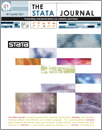Home  >>  Archives  >>  Volume 11 Number 4  >>  st0239

#### The Stata JournalVolume 11 Number 4: pp. 518-544

Subscribe to the Stata Journal## Bayesian model averaging and weighted-average least squares: Equivariance, stability, and numerical issues

 Giuseppe De Luca ISFOL Rome, Italy g.deluca@isfol.it Jan R. Magnus Tilburg University Tilburg, The Netherlands magnus@uvt.nl
Abstract.  In this article, we describe the estimation of linear regression models with uncertainty about the choice of the explanatory variables. We introduce the Stata commands bma and wals, which implement, respectively, the exact Bayesian model-averaging estimator and the weighted-average least-squares estimator developed by Magnus, Powell, and Pr¨ufer (2010, Journal of Econometrics 154: 139–153). Unlike standard pretest estimators that are based on some preliminary diagnostic test, these model-averaging estimators provide a coherent way of making inference on the regression parameters of interest by taking into account the uncertainty due to both the estimation and the model selection steps. Special emphasis is given to several practical issues that users are likely to face in applied work: equivariance to certain transformations of the explanatory variables, stability, accuracy, computing speed, and out-of-memory problems. Performances of our bma and wals commands are illustrated using simulated data and empirical applications from the literature on model-averaging estimation.

View all articles by these authors: Giuseppe De Luca, Jan R. Magnus

View all articles with these keywords: bma, wals, model uncertainty, model averaging, Bayesian analysis, exact Bayesian model averaging, weighted-average least squares